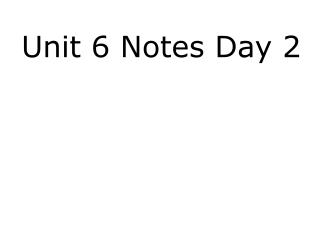DownloadDownload PresentationUnit 6 Notes Day 2

Unit 6 Notes Day 2

Télécharger la présentationUnit 6 Notes Day 2

- - - - - - - - - - - - - - - - - - - - - - - - - - - E N D - - - - - - - - - - - - - - - - - - - - - - - - - - -
Presentation Transcript

1. Unit 6 Notes Day 2

2. A correspondence is a way of matching up two sets of objects. If two polygons are congruent, all of their corresponding sides and angles are congruent. In a congruence statement, the vertices in the second polygon are written in order of correspondence with the first polygon.

3. WX @ KL a + 8 = 24 –8 –8 a = 16 Example 1 In the figure, quadrilateral VWXY@ quadrilateral JKLM. Find a. Subtract 8 from both sides.

4. ML @ YX 6b = 30 6b = 30 6 6 Example 2 In the figure, quadrilateral VWXY@ quadrilateral JKLM. Find b. Divide both sides by 6. b = 5

5. J @V 5c = 85 5c = 85 5 5 Example 3 In the figure, quadrilateral VWXY@ quadrilateral JKLM. Find c. Divide both sides by 5. c = 17

6. IH @ RS 3a = 6 3a = 6 3 3 Example 4 In the figure, quadrilateral JIHK@ quadrilateral QRST. Find a. Divide both sides by 3. 3a I H a = 2 6 4b° S R 120° J 30° Q K c + 10° T

7. H @S 4b = 120 4b = 120 4 4 Example 5 In the figure, quadrilateral JIHK@ quadrilateral QRST. Find b. Divide both sides by 4. 3a I H b = 30° 6 4b° S R 120° J 30° Q K c + 10° T

8. K @T c + 10 = 30 c + 10 = 30 –10 –10 Example 6 In the figure, quadrilateral JIHK@ quadrilateral QRST. Find c. Subtract 10 from both sides. 3a I H c = 20° 6 90° 4b° S R 120° 90° J 30° c + 10° Q K T

9. 1. Find XY. 3. Find CD. Example 7 In the figure, WXYZ@ABCD 10 80° 2. Find mB. 8 90° 4. Find mZ.